# Angol nyelvű matematika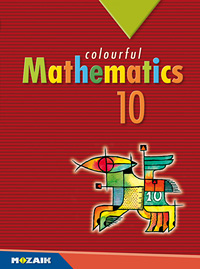MS-6310

## Colourful Mathematics 10.

10. évfolyam, 2. kiadás (2017. 08. 22.)
Mozaik Kiadó
méret: 170x240 mm
terjedelem: 256 oldal
tanterv: NAT 2012
4 980 Ft
3 984 Ft (20% osztálykedvezménnyel)*
Kosárba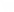* Legalább 15 példány megrendelése esetén 20% kedvezményt tudunk biztosítani 2021.03.31 és 2021.10.15 között.
 A kiadvány az MS-2310 Sokszínű matematika 10. c. kötet angol nyelvű változata. This book is the English version of the Hungarian market leader textbook titled Sokszínű matematika 10.
Kapcsolódó kiadványok
 A kiadvány digitális változata a könyvben levő kóddal ingyenesen elérhető*A kiadvány hátsó borítójának belső oldalán található egyedi kóddal a kiadvány digitálisan is elérhető. Az aktivált kódokkal DÍJMENTES hozzáférést biztosítunk a kiadvány mozaWeb Home változatához az aktiválástól számított minimum egy éves időtartamra. A kódok csak egyszer aktiválhatók.
Mintaoldalak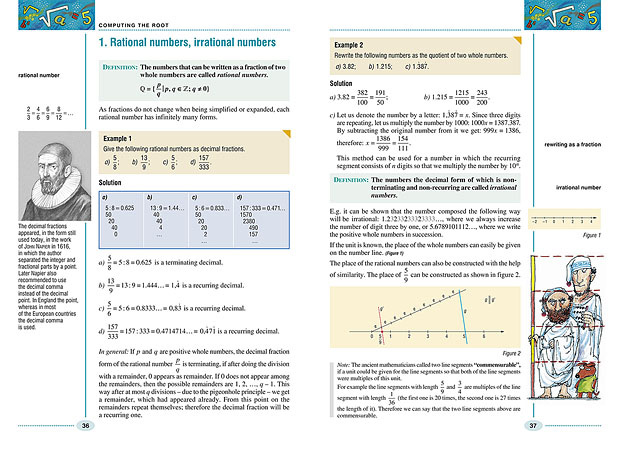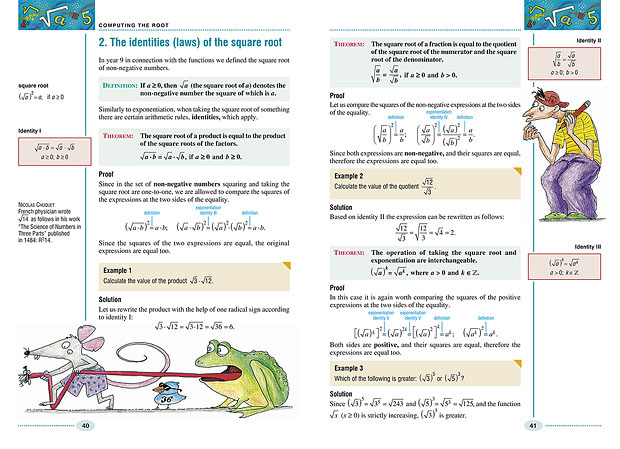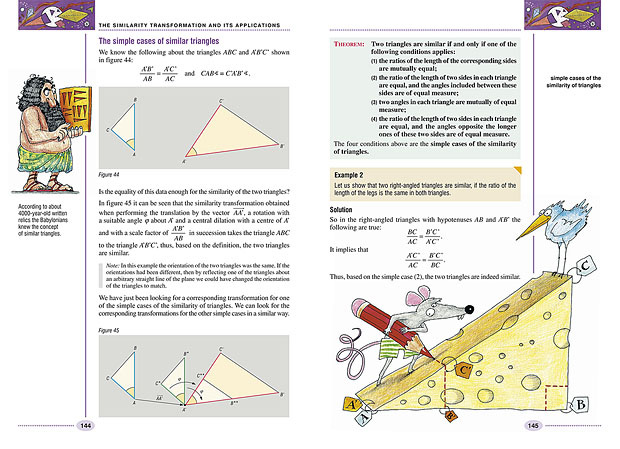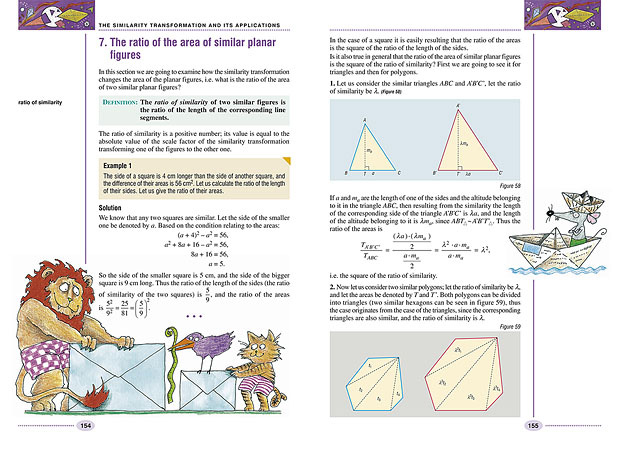Tartalomjegyzék
 Mathematical reasoning 10 1. What does it imply? 10 2. The pigeonhole principle 21 3. Arrangement (ordering) problems 29 4. Picking problems 32 Computing the root 36 1. Rational numbers, irrational numbers 36 2. The identities (laws) of the square root 40 3. Applying the identities (laws) of the square root 44 4. The nth root of numbers 50 5. The identities (laws) of the nth root . 53 The quadratic equation 60 1. The quadratic equation and function 60 2. The quadratic formula 64 3. The zero product form. The relation between the roots and the coefficients 69 4. Equations of higher degree which can be reduced to quadratic equations 74 5. Quadratic inequalities 80 6. Parametric quadratic equations (higher level courseware) 84 7. Equations involving square roots 90 8. Quadratic simultaneous equations 96 9. Arithmetic and geometric mean 101 10. Extreme value exercises (higher level courseware) 106 11. Problems leading to quadratic equations 110 Geometry 116 Widening the knowledge about circles 116 1. Reminder 116 2. The theorem of the central and inscribed/tangent-chord angles 117 3. The theorem of inscribed angles; the arc of viewing angles 121 4. The theorem of inscribed quadrilaterals (higher level courseware) 125 The similarity transformation and its applications 129 1. Parallel intercepting lines, parallel intercepting line segments (higher level courseware) 129 2. The angle bisector theorem (higher level courseware) 135 3. The transformation of central dilation (or homothety) 137 4. The similarity transformation 141 5. Similarity of figures; the simple cases of similar triangles 143 6. A few applications of similarity 147 7. The ratio of the area of similar planar figures 154 8. The ratio of the volume of similar solids 158 Trigonometric functions of acute angles 161 1. Determining distances with the help of similarity 161 2. Trigonometric functions of acute angles 164 3. Relations between the trigonometric functions of acute angles 168 4. Trigonometric functions of special angles 172 5. Determining several data of a triangle with the help of trigonometric functions 175 6. Calculations in the plane and in space with the help of trigonometric functions 180 Vectors 184 1. The concept of a vector; the sum and the difference of vectors; scalar multiplication of vectors (reminder) 184 2. Expressing vectors as the sum of components in different directions 188 3. Applying vectors in the plane and in space 194 4. Vectors in the coordinate system, the coordinates of a vector, operations with vectors given with coordinates 199 Trigonometric functions 204 1. The definition and the simple properties of the sine and the cosine function 204 2. The graph of the sine function 209 3. The graph of the cosine function, equations, inequalities 214 4. The tangent and the cotangent function 221 5. Compound exercises and applications . 228 6. Geometric applications 232 Calculation of probability 238 1. Events 238 2. Operations with events 243 3. Experiments, frequency, relative frequency, probability 248 4. The classical model of probability .. 251
A kiadvány bevezetője
 Guide to use the course book. The notations and highlights used in the book help with acquiring the courseware. - The train of thought of the worked examples show samples how to understand the methods and processes and how to solve the subsequent exercises. - The most important definitions and theorems are denoted by colourful highlights. - The parts of the courseware in small print and the worked examples noted in claret colour help with deeper understanding of the courseware. These pieces of knowledge are necessary for the higher level of graduation. - Figures, the key points of the given lesson, review and explanatory parts along with interesting facts of the history of mathematics can be found on the margin. The difficulty level of the examples and the appointed exercises is denoted by three different colours: Yellow: drilling exercises with basic level difficulty; the solution and drilling of these exercises is essential for the progress. Blue: exercises the difficulty of which corresponds to the intermediate level of graduation. Claret: problems and exercises that help with preparing for the higher level of graduation. These colour codes correspond to the notations used in the Colourful mathematics workbooks of Mozaik Education. The workbook series contains more than 3000 exercises which are suitable for drilling, working on in lessons and which help with preparing for the graduation. The end results of the appointed exercises can be found on the following website: www.mozaik.info.hu. Website www.mozaweb.hu offers more help material for processing with the course book.

© MOZAIK KIADÓ - SZEGED, 2021 | Mobil